Transpose of a matrix

Chapter 3 Class 12 Matrices
Concept wise

In transpose of a matrix,

• Rows become columns and,
• Column become rows

For matrix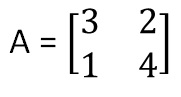It’s transpose is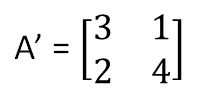We denote it by A’

Similarly for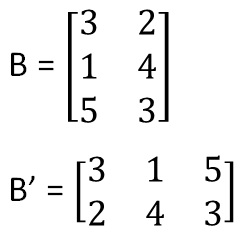Let’s look at some properties of transpose

## Properties of transpose of a matrix

• (A’)’ = A
• (kA)’ = kA’
• (A + B)’ = A’ + B’
• (AB)’ = B’ A’

Let’s try to prove them one by one

Let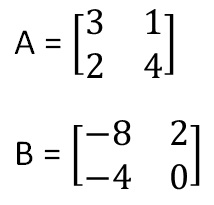(A’)’ = A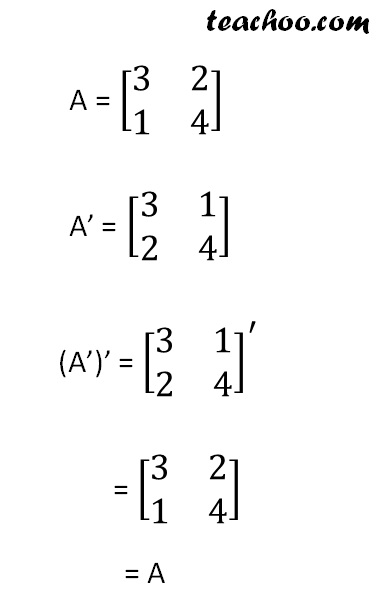Therefore,

(A’)’ = A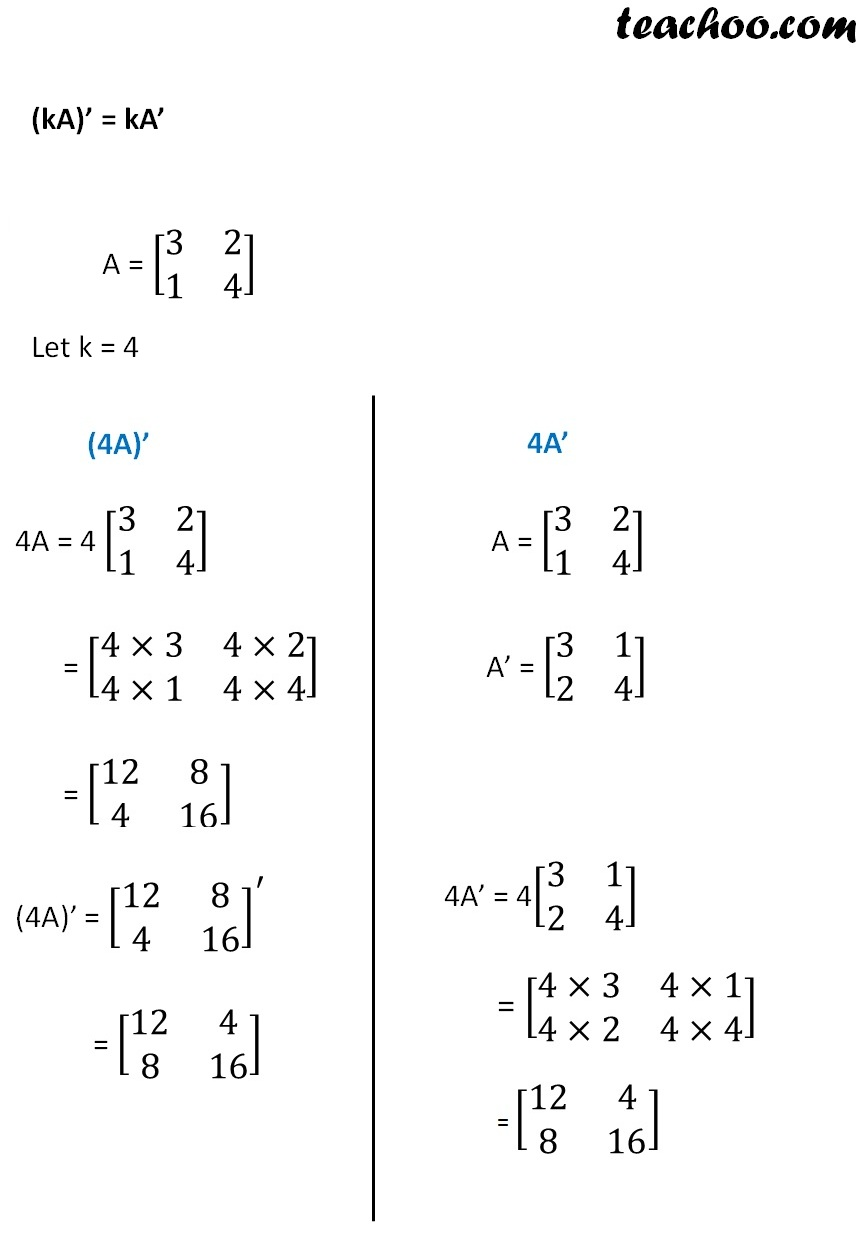Therefore,

(4A)’ = 4A’

## (A + B)’ = A’ + B’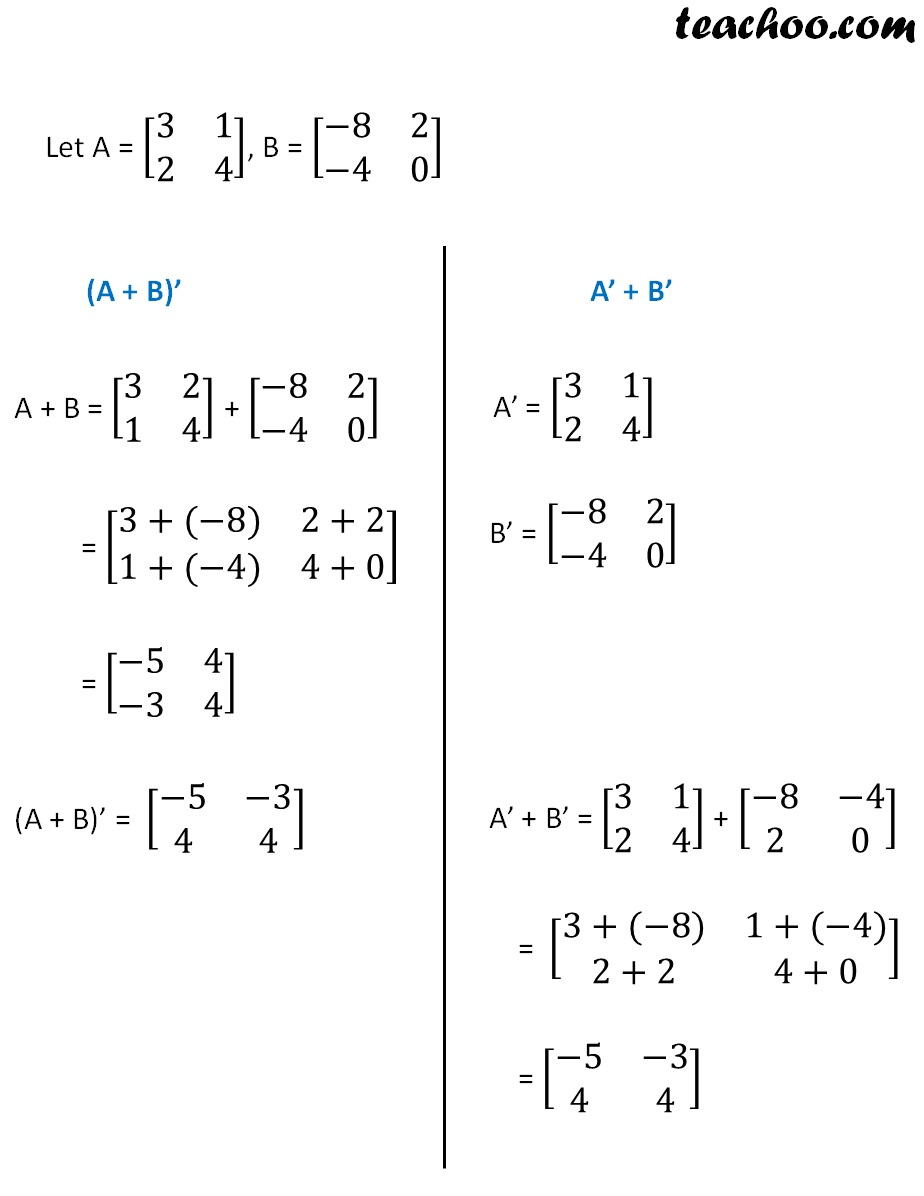## (AB)’ = B’ A’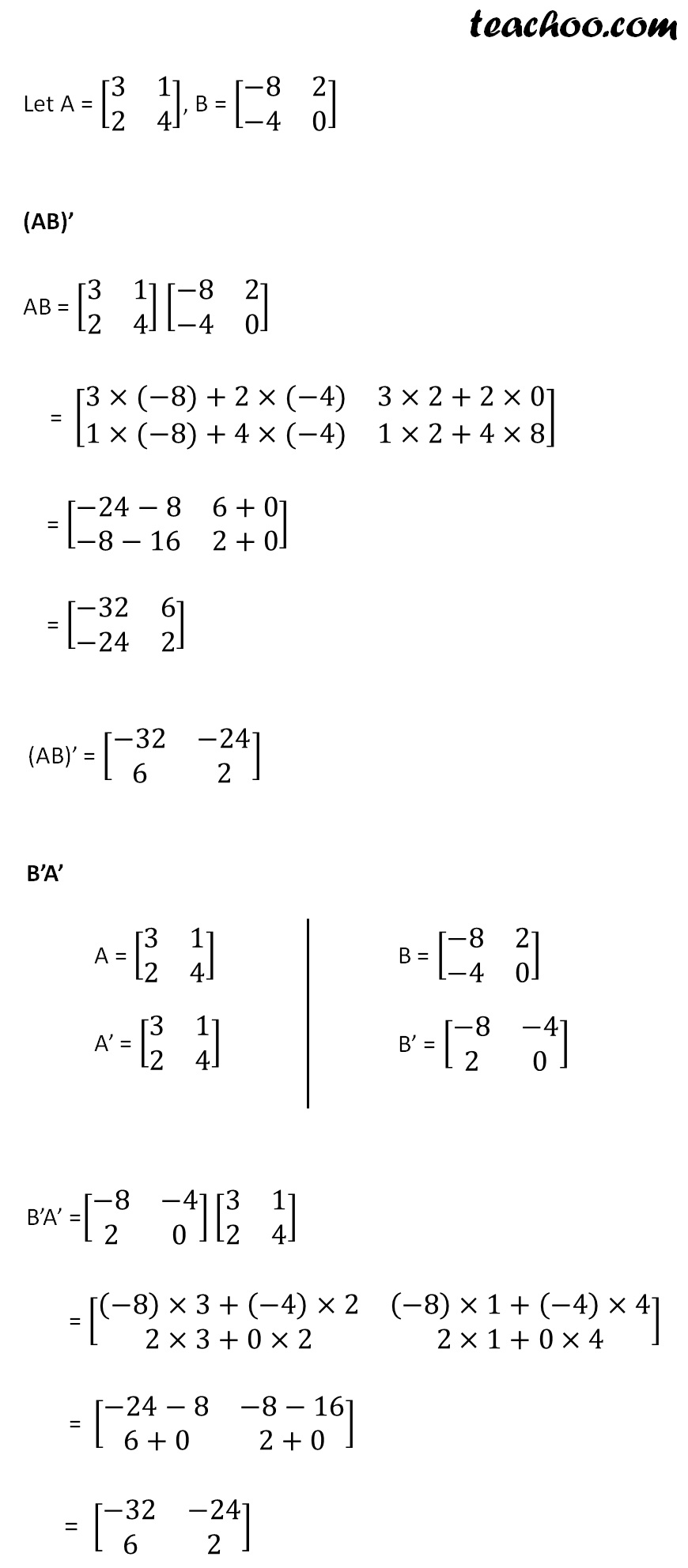Therefore,

(AB)’ = B’ A’

Learn in your speed, with individual attention - Teachoo Maths 1-on-1 Class

### Transcript

For matrix A = [■8(3&2@1&4)] It’s transpose is A’ = [■8(3&1@2&4)] B = [■8(3&2@1&4@5&3)] B’ = [■8(3&1&5@2&4&3)] A = [■8(3&1@2&4)] B = [■8(−8&2@−4&0)] A = [■8(3&2@1&4)] A’ = [■8(3&1@2&4)] (A’)’ = [■8(3&1@2&4)]^′ = [■8(3&2@1&4)] = A A = [■8(3&2@1&4)] Let k = 4 (4A)’ 4A = 4 [■8(3&2@1&4)] = [■8(4×3&4×2@4×1&4×4)] = [■8(12&8@4&16)] 4A’ A = [■8(3&2@1&4)] A’ = [■8(3&1@2&4)] (4A)’ = [■8(12&8@4&16)]^′ = [■8(12&4@8&16)] 4A’ = 4[■8(3&1@2&4)] = [■8(4×3&4×1@4×2&4×4)] = [■8(12&4@8&16)] Let A = [■8(3&1@2&4)], B = [■8(−8&2@−4&0)] (A + B)’ A + B = [■8(3&2@1&4)] + [■8(−8&2@−4&0)] = [■8(3+(−8)&2+2@1+(−4)&4+0)] = [■8(−5&4@−3&4)] A’ + B’ A’ = [■8(3&1@2&4)] B’ = [■8(−8&2@−4&0)] (A + B)’ = [■8(−5&−3@4&4)] A’ + B’ = [■8(3&1@2&4)] + [■8(−8&−4@2&0)] = [■8(3+(−8)&1+(−4)@2+2&4+0)] = [■8(−5&−3@4&4)] Let A = [■8(3&1@2&4)], B = [■8(−8&2@−4&0)] (AB)’ AB = [■8(3&1@2&4)] [■8(−8&2@−4&0)] = [■8(3×(−8)+2×(−4)&3×2+2×0@1×(−8)+4×(−4)&1×2+4×8)] = [■8(−24−8&6+0@−8−16&2+0)] = [■8(−32&6@−24&2)] (AB)’ = [■8(−32&−24@6&2)] B’A’ A = [■8(3&1@2&4)] A’ = [■8(3&1@2&4)] B = [■8(−8&2@−4&0)] B’ = [■8(−8&−4@2&0)] B’A’ =[■8(−8&−4@2&0)][■8(3&1@2&4)] = [■8((−8)×3+(−4)×2&(−8)×1+(−4)×4@2×3+0×2&2×1+0×4)] = [■8(−24−8&−8−16@6+0&2+0)] = [■8(−32&−24@6&2)]